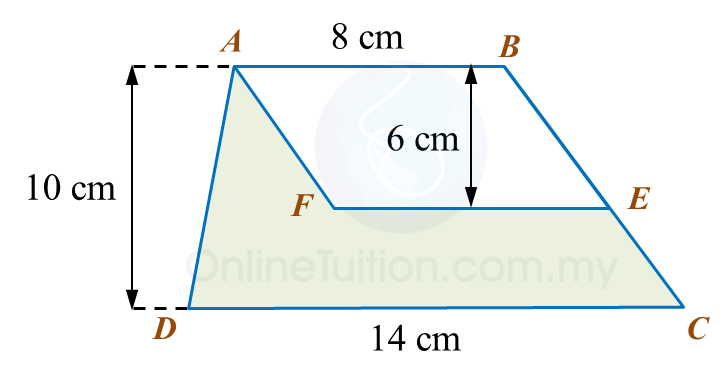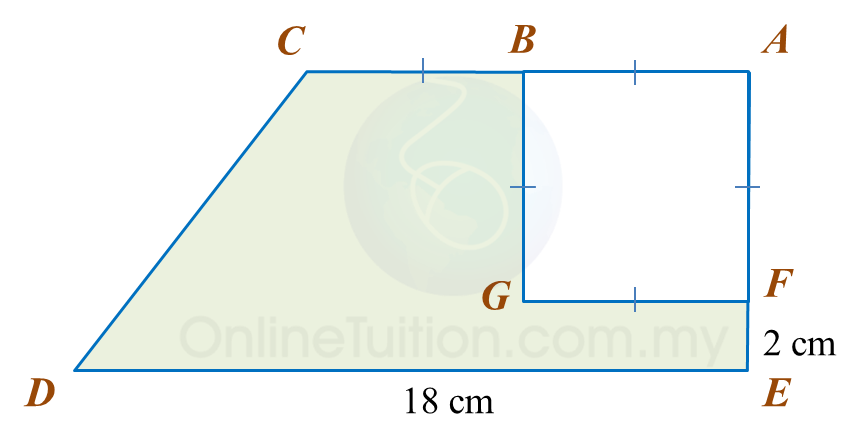# 11.2.1 Perimeter and Area, PT3 Practice

Question 1:
In the diagram, ABCD is a trapezium and ABEF is a parallelogram.Calculate the area, in cm2, of the coloured region.

Solution
:

Question 2:
Diagram below shows a rectangle ABCD.Calculate the area, in cm2, of the coloured region.

Solution:

Question 3:
In diagram below, AEC is a right-angled triangle with an area of 54 cm2 and BCDF is a rectangle.Calculate
(a) the perimeter, in cm, of the coloured region.
(b) the area, in cm2, of the coloured region.

Solution:

Question 4:
Diagram below shows a trapezium ABCDE. ABGF is a square with an area of 36 cm2.Calculate
(a) the perimeter, in cm, of the coloured region.
(b) the area, in cm2, of the coloured region.

Solution: